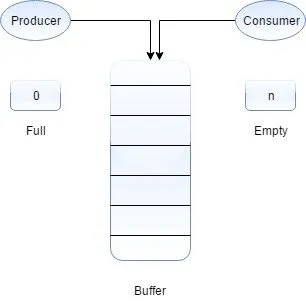# Networking - C Classic Synchronization Problems in LinuxHello my friends it's me again! Today we will get into Classic Synchronization Problems and how we solve them. We will talk about the Producer-Consumer, Reader-Writer and Dining Philosophers Problems in C Linux. I will suppose that you have seen the posts about Synchronization and IPC. So, let's get started!

# Producer-Consumer Problem:

This problem is all about Processes/Threads that produce/consume Data that is inside of a in-between storage. So, we have 2 basic operations: put() and get(). We can't get an item if the storage is empty and we can't add an item if we reached the maximum storage capacity. The last is true only if our storage has limited capacity and not infinite. So, the Consumer must be blocked when the storage is empty and the Producer in the other hand must be blocked if the storage is full.

To solve this problem we will use 2 general usage semaphores that count the empty and full slots, cause thats exactly how a semaphore works! If the access is a critical section (that it mostly will be) we will also have to block the Producers and Consumers when another Producer or Consumer is already putting or getting a Item. So, we will also use a mutex or minary semaphore

So, finally the Producer and Consumer Code look contains:

Semaphores:

``sem_t full, empty, mutex;``
``sem_init(&full, 0, 0);``
``sem_init(&empty, 0, N);``
``sem_init(&mutex, 0, 1);  ``

Producer:

``    // produce item``
``    sem_wait(&empty);``
``    sem_wait(&mutex);``
``    // put item``
``    sem_post(&mutex);``
``    sem_post(&full);``

Consumer:

``    sem_wait(&full);``
``    sem_wait(&mutex);``
``    // get item``
``    sem_post(&mutex);``
``    sem_post(&empty);``

So, we will need a mutex to have either all Readers or a Writer get access. We will also have 2 mutexes for the read or write queues. We will also store 2 counters with number of Writers and Readers waiting so that the Readers get blocked if we have a blocked Writer, and also have 2 counters with number of Writers or Readers in the Critical Section

Finally our Code will contain the following:

Semaphores and Counters:

``sem_t wq, rq, mutex;``
``sem_init(&wq, 0, 0);``
``sem_init(&rq, 0, 0);``
``sem_init(&mutex, 0, 1);  ``
``int ww=0, rw=0; // waiting``
``int wrs=0, rds=0; // in section``

Writer:

``sem_wait(&mutex);``
``if (rds+wrs > 0) {``
``    ww++;``
``    sem_post(&mutex);``
``    sem_wait(&wq);``
``}``
``else {``
``    wrs++;``
``    sem_post(&mutex);``
``}``
``// write ``
``sem_wait(&mutex);``
``wrs--;``
``if (rw > 0) {``
``    rw--; ``
``    rds++;``
``    sem_post(&rq);``
``}``
``else {``
``    if (ww > 0) {``
``        ww--;``
``        wrs++;``
``        sem_post(&wq);``
``    }``
``    sem_post(&mutex);``
``}``

``sem_wait(&mutex);``
``if (wrs+ww > 0) {``
``    rw++;``
``    sem_post(&mutex);``
``    sem_wait(&rq);``
``    if (rw > 0) {``
``        rw--;``
``        rds++;``
``        sem_post(&rq);``
``    }``
``    else {``
``        sem_post(&mutex);``
``    }``
``}``
``else {``
``    rds++;``
``    sem_post(&mutex);``
``}``
``// read``
``sem_wait(&mutex);``
``rds--;``
``if (rds == 0) && (ww > 0) {``
``    ww--;``
``    wrs++;``
``    sem_post(&wq);``
``}``
``sem_post(&mutex);``

# Dining Philosophers Problem:

The lifecycle of a Philosopher has 3 phases: thinking, being hungry, eating. We suppose that we have N philosophers on a circular table, but also N plates of food and N forks. So, a philosopher must grab the left and right forks to eat! We have a problem, cause we may end up with all philosophers having 1 fork and so we need some kind of control and synchronization. So, each fork is a Critical Section between 2 neighbour philosophers

Suppose that each fork is a mutex and that we get a fork by using up() and let it down by using down(). That way the 2 philosophers can't get the same fork, but we end up with another problem. Having all philosophers use the same Code and get hungry the same time, all would get their left or right fork (depending on the Code) and so all would have a single fork and we would end up with a deadlock

To fix this second problem we can use 2 Solutions. We could allow only N-1 of the philosophers have access on the table. We could break the symmetry by having one or more of the philosophers take the other fork first. in the first solution we will have a general semaphore that starts counting down from N-1 and in the second solution we will simply use a different code or a if/else statement for 1 or more philoshopers to break the symmetry.

So, our Code uses the following:

Semaphore in both:

``sem_t fork[N];``
``for(i=0; i<N; i++) sem_init(&fork[i], 0 , 1);``

Semaphore for first solution:

``sem_t table;``
``sem_init(&table, 0, N-1);``

First solution:

``int i; // philosopher index``
``while (1) {``
``    // thinking``
``    // hungry``
``    sem_wait(&table);``
``    sem_wait(&fork[RIGHT(i)]);``
``    sem_wait(&fork[LEFT(i)]);``
``    // eating``
``    sem_post(&fork[RIGHT(i)]);``
``    sem_post(&fork[LEFT(i)]);``
``    sem_post(&table);``
``}``

Second solution:

``int i; // philosopher index``
``while (1) {``
``    // thinking``
``    if (i != N-1) {``
``        sem_wait(&fork[RIGHT(i)]);``
``        sem_wait(&fork[LEFT(i)]);``
``    }``
``    else {``
``         sem_wait(&fork[LEFT(i)]);``
``         sem_wait(&fork[RIGHT(i)]);``
``    }``
``    // eating``
``    sem_post(&fork[RIGHT(i)]);``
``    sem_post&fork[LEFT(i)]);``
``}``

Where right() and left() will be functions that return the left and right index!

``int RIGHT(int index){``
``    if(index == N-1){``
``        return 0;``
``    }``
``    return index++;``
``}``
``int LEFT(int index){``
``    if(index == 0){``
``        return N-1;``
``    }``
``    return index--;``
``}``

And this is actually it. Using these basic structures you can solve any Synchronization problem and I hope that you enjoyed this post!

Next time we will implement threads and synchronization in Java and after that we will get into "real" network programming starting off with Sockets!

Until next time...Bye!

H2
H3
H4
3 columns
2 columns
1 column